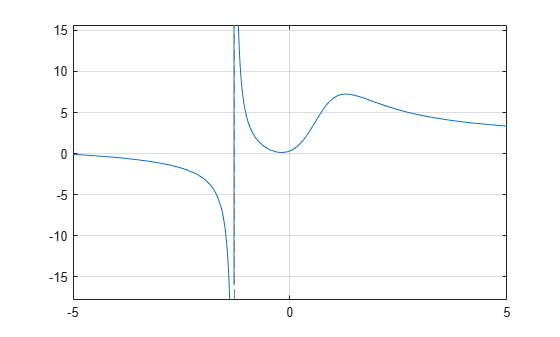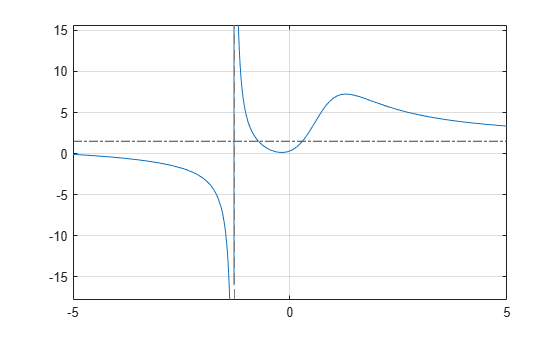Main Content

# Differentiation

This example shows how to analytically find and evaluate derivatives using Symbolic Math Toolbox™. In the example you will find the 1st and 2nd derivative of f(x) and use these derivatives to find local maxima, minima and inflection points.### First Derivatives: Finding Local Minima and Maxima

Computing the first derivative of an expression helps you find local minima and maxima of that expression. Before creating a symbolic expression, declare symbolic variables:

`syms x`

By default, solutions that include imaginary components are included in the results. Here, consider only real values of `x` by setting the assumption that `x` is real:

`assume(x, 'real')`

As an example, create a rational expression (i.e., a fraction where the numerator and denominator are polynomial expressions).

`f = (3*x^3 + 17*x^2 + 6*x + 1)/(2*x^3 - x + 3)`
```f =  $\frac{3 {x}^{3}+17 {x}^{2}+6 x+1}{2 {x}^{3}-x+3}$```

Plotting this expression shows that the expression has horizontal and vertical asymptotes, a local minimum between -1 and 0, and a local maximum between 1 and 2:

```fplot(f) grid```To find the horizontal asymptote, compute the limits of `f` for `x` approaching positive and negative infinities. The horizontal asymptote is `y = 3/2`:

`lim_left = limit(f, x, -inf)`
```lim_left =  $\frac{3}{2}$```
`lim_right = limit(f, x, inf)`
```lim_right =  $\frac{3}{2}$```

Add this horizontal asymptote to the plot:

```hold on plot(xlim, [lim_right lim_right], 'LineStyle', '-.', 'Color', [0.25 0.25 0.25])```To find the vertical asymptote of `f`, find the poles of `f`:

`pole_pos = poles(f, x)`
```pole_pos =  $-\frac{1}{6 {\left(\frac{3}{4}-\frac{\sqrt{241} \sqrt{432}}{432}\right)}^{1/3}}-{\left(\frac{3}{4}-\frac{\sqrt{241} \sqrt{432}}{432}\right)}^{1/3}$```

Approximate the exact solution numerically by using the `double` function:

`double(pole_pos)`
```ans = -1.2896 ```

Now find the local minimum and maximum of `f`. If a point is a local extremum (either minimum or maximum), the first derivative of the expression at that point is equal to zero. Compute the derivative of `f` using `diff`:

`g = diff(f, x)`
```g =  $\frac{9 {x}^{2}+34 x+6}{2 {x}^{3}-x+3}-\frac{\left(6 {x}^{2}-1\right) \left(3 {x}^{3}+17 {x}^{2}+6 x+1\right)}{{\left(2 {x}^{3}-x+3\right)}^{2}}$```

To find the local extrema of `f`, solve the equation `g == 0`:

`g0 = solve(g, x)`
```g0 =  ```

Approximate the exact solution numerically by using the `double` function:

`double(g0)`
```ans = 2×1 -0.1892 1.2860 ```

The expression `f` has a local maximum at `x = 1.286` and a local minimum at `x = -0.189`. Obtain the function values at these points using `subs`:

`f0 = subs(f,x,g0)`
```f0 =  ```

Approximate the exact solution numerically by using the `double` function on the variable `f0`:

`double(f0)`
```ans = 2×1 0.1427 7.2410 ```

Add point markers to the graph at the extrema:

`plot(g0, f0, 'ok')`### Second Derivatives: Finding Inflection Points

Computing the second derivative lets you find inflection points of the expression. The most efficient way to compute second or higher-order derivatives is to use the parameter that specifies the order of the derivative:

`h = diff(f, x, 2)`
```h =  ```

Now Simplify that result:

`h = simplify(h)`
```h =  $\frac{2 \left(68 {x}^{6}+90 {x}^{5}+18 {x}^{4}-699 {x}^{3}-249 {x}^{2}+63 x+172\right)}{{\left(2 {x}^{3}-x+3\right)}^{3}}$```

To find inflection points of `f`, solve the equation `h = 0`. Here, use the numeric solver `vpasolve` to calculate floating-point approximations of the solutions:

`h0 = vpasolve(h, x)`
```h0 =  $\left(\begin{array}{c}0.57871842655441748319601085860196\\ 1.8651543689917122385037075917613\\ -1.4228127856020972275345064554049-1.8180342567480118987898749770461 \mathrm{i}\\ -1.4228127856020972275345064554049+1.8180342567480118987898749770461 \mathrm{i}\\ -0.46088831805332057449182335801198+0.47672261854520359440077796751805 \mathrm{i}\\ -0.46088831805332057449182335801198-0.47672261854520359440077796751805 \mathrm{i}\end{array}\right)$```

The expression `f` has two inflection points: `x = 1.865` and `x = 0.579`. Note that `vpasolve` also returns complex solutions. Discard those:

`h0(imag(h0)~=0) = []`
```h0 =  $\left(\begin{array}{c}0.57871842655441748319601085860196\\ 1.8651543689917122385037075917613\end{array}\right)$```

Add markers to the plot showing the inflection points:

```plot(h0, subs(f,x,h0), '*k') hold off```## Support

#### Mathematical Modeling with Symbolic Math Toolbox

Get examples and videos